# Supervised Learning

In this chapter, we are going to discuss Supervised Learning. I think this is the most common machine learning algorithm. I would start with an example and hope it will be cleared later. Let’s say we are going to predict housing prices. I take this picture example from Andrew Ng Coursera.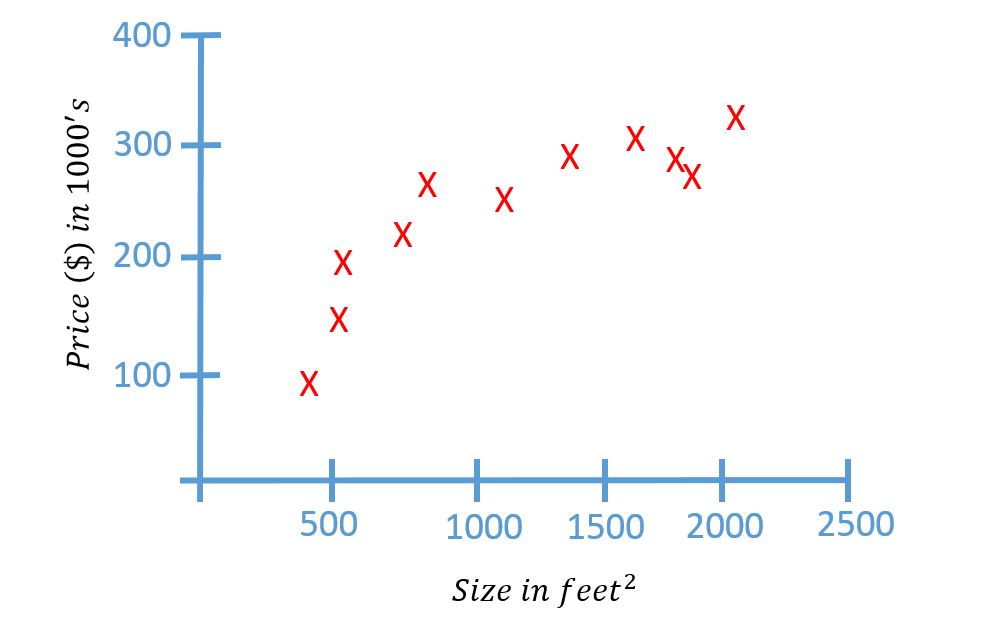Here on the horizontal axes is the size of different houses and on the vertical axis, the price of different houses.

Given this data, let’s say I have a friend who owns $$750 feet^2$$ and He wants to sell the house and I want to know how much I can get for the house. So how the learning algorithm help me in this case? The learning algorithm probably can come out with a straight line through the data and based on that, it looks like maybe the house can be sold for $150,000. But this is not the only output (model) learning algorithm can give.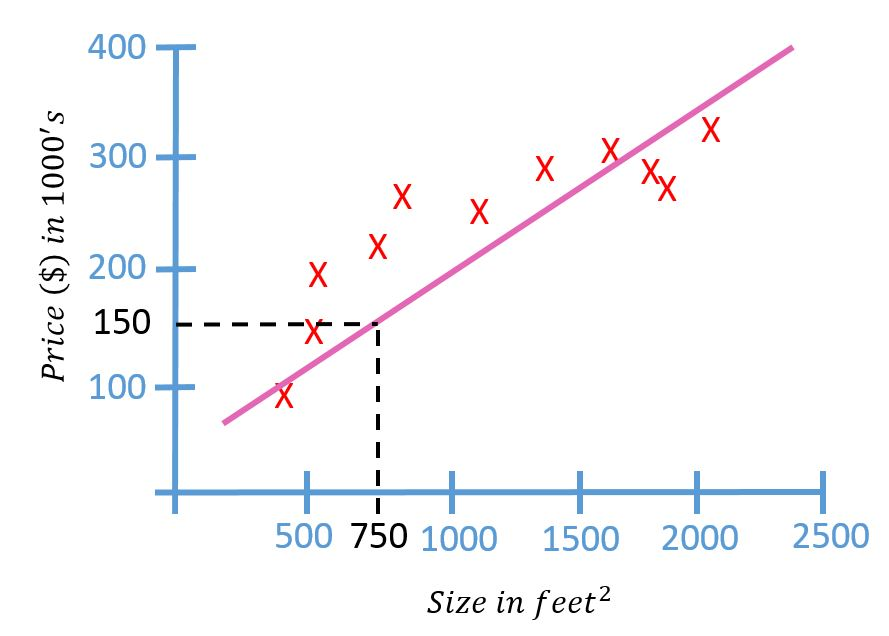There might be a better one. for example, instead of the straight line to the data, we can use a quadratic function (second-order polynomial) to fit the data. And probably the result look like this picture below.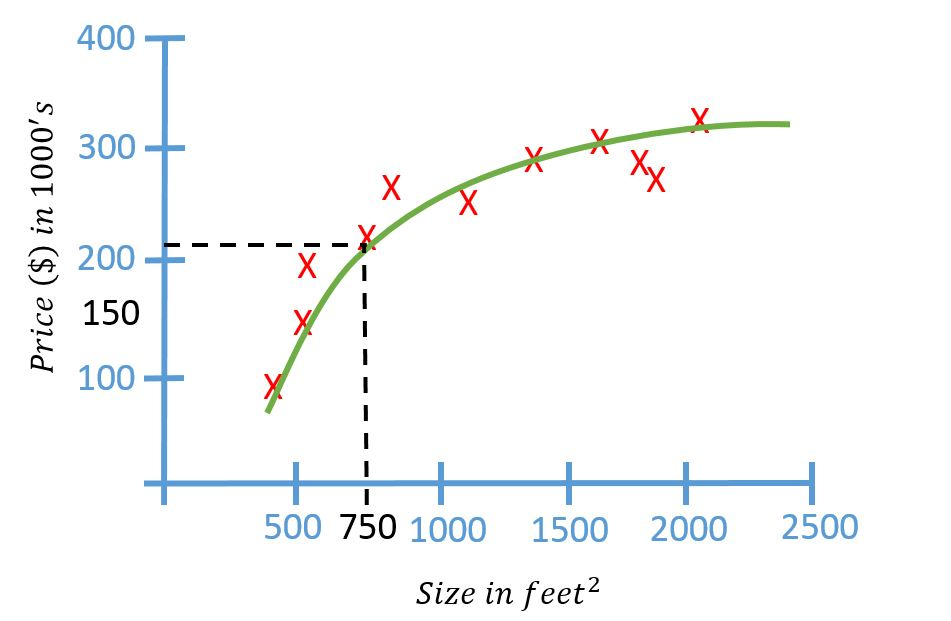The prediction is slightly different with the straight line. In here the house’s price around$200,000. one thing we are going to discuss later is how to decide, do we want to fit a straight line to the data or use a quadratic function.
This is an example of a supervised learning algorithm. The term “supervised learning” refers to the fact that we gave the algorithm a data set, in which the “right answers” (later we call label/ground truth) were given. Remember, We gave dataset of houses in which for every example in this data set, we told it what is the right price that the house sold for. The purpose of the algorithm was to just produce more of these right answers such as for my friend house ($$750 feet^2$$, prediction $200,000). In Machine Learning, this is also called a regression problem. In Regression problem, we are trying to predict a continuous value output. in this case the price. In the previous case, we only use one feature or one attribute. But actually, it can be more than one. Let’s say we have price and size of the houses. we are going to make a system that will decide whether we will buy or not. In that case, maybe our data set will look like this.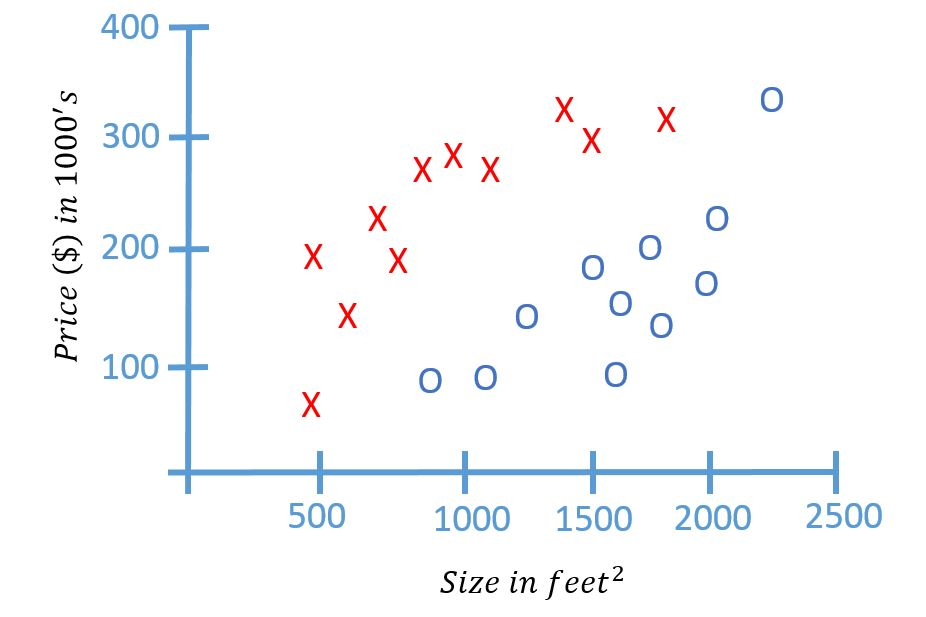In this data set the red cross means data price and size of the houses that we are not going to buy and the blue circle means, the houses that we are going to buy. By given the dataset like this, what the learning algorithm might do us throw the straight line through the data to try to separate out. For example the algorithm with throw the straight line like this picture to separate out whether to buy or not.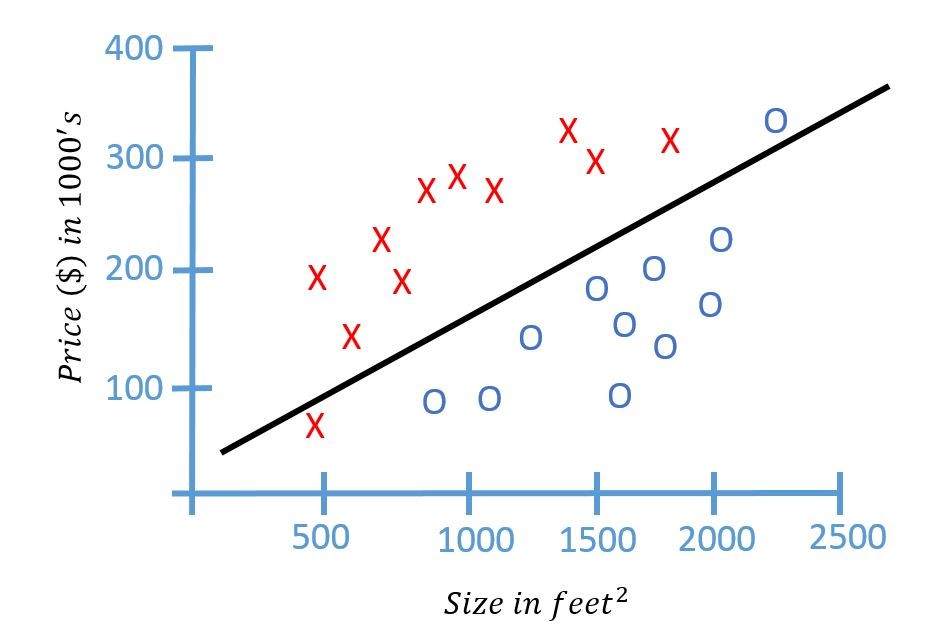Then, if there is a new house that is offered for sale, we can plot the new house into our model. let’s say the house is$200,000 for $$1200 feet^2$$. if we plot the data it would be like this,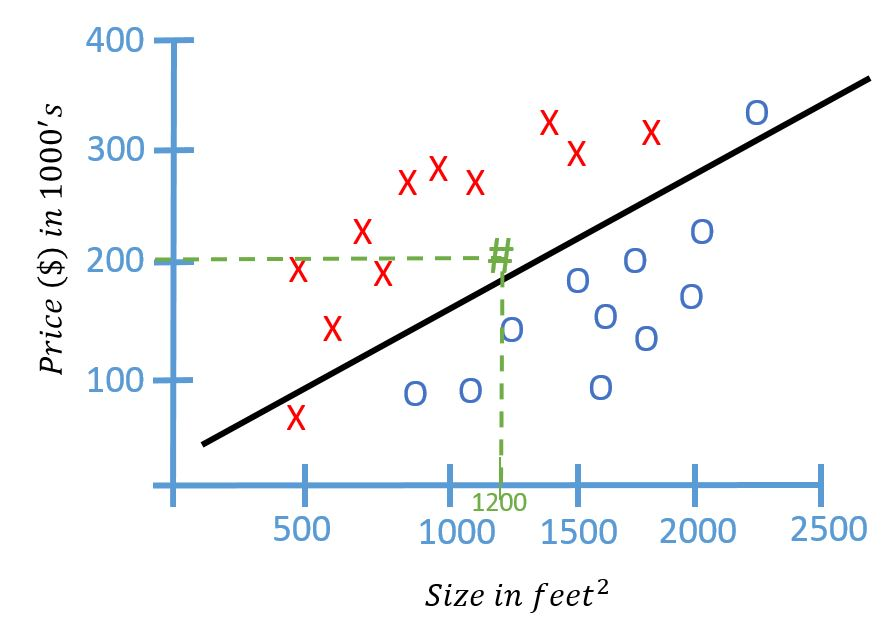By this result, very likely we are not going to buy this house. In this example, we had two features. namely the price and the size, of the house. In other machine learning problem, we will often have more features. This particular example problem is called classification problem (binary classification for a specific name).

# Introduction to Machine Learning

Welcome to my machine learning notes. Machine learning is getting popular nowadays. On this notes, we learn about machine learning algorithms. I wish this notes would be useful.

we probably use a machine learning algorithm every day without realizing. For example, every time we use a search engine to search on the internet, it is machine learning. another example, when we use Facebook and it recognizes someone in the photo that is also machine learning. Machine Learning is set of algorithms that try to mimic how the human brain learns.

I believe Machine Learning still a part of artificial intelligence (AI) or the extension from AI. Why machine learning so popular today? I think it because machine learning gives new capability of the computer and it touches many aspects of industry and life. For my self, I am learning machine learning for computer vision. But, I would tell you that machine learning is not limited only to computer vision. we can use machine learning in many other fields.

Here some examples of machine learning.
1. Data Mining
This can be in many fields, such as web click data, medical records, or even biology. For example, Amazon has tons of purchasing data. They want to understand the users better and to serve the users better by providing recommendation system. On medical fields, for example, the hospital wants to make a support system by inputting the symptoms to recognize the disease.2. Applications can’t program by hand
For example, handwriting recognition. We just didn’t know how to tell the computer to recognize handwriting characters or numbers. the only thing that worked was having computer learn by itself how to recognize handwriting character by giving some examples.3. Computer Vision (Object Recognition)
This is similar to the handwriting recognition. For example, we want to make a computer recognize some objects. We don’t know how to tell the computer to recognize car for example. we just simply feed the computer car pictures and lets the computer learn itself.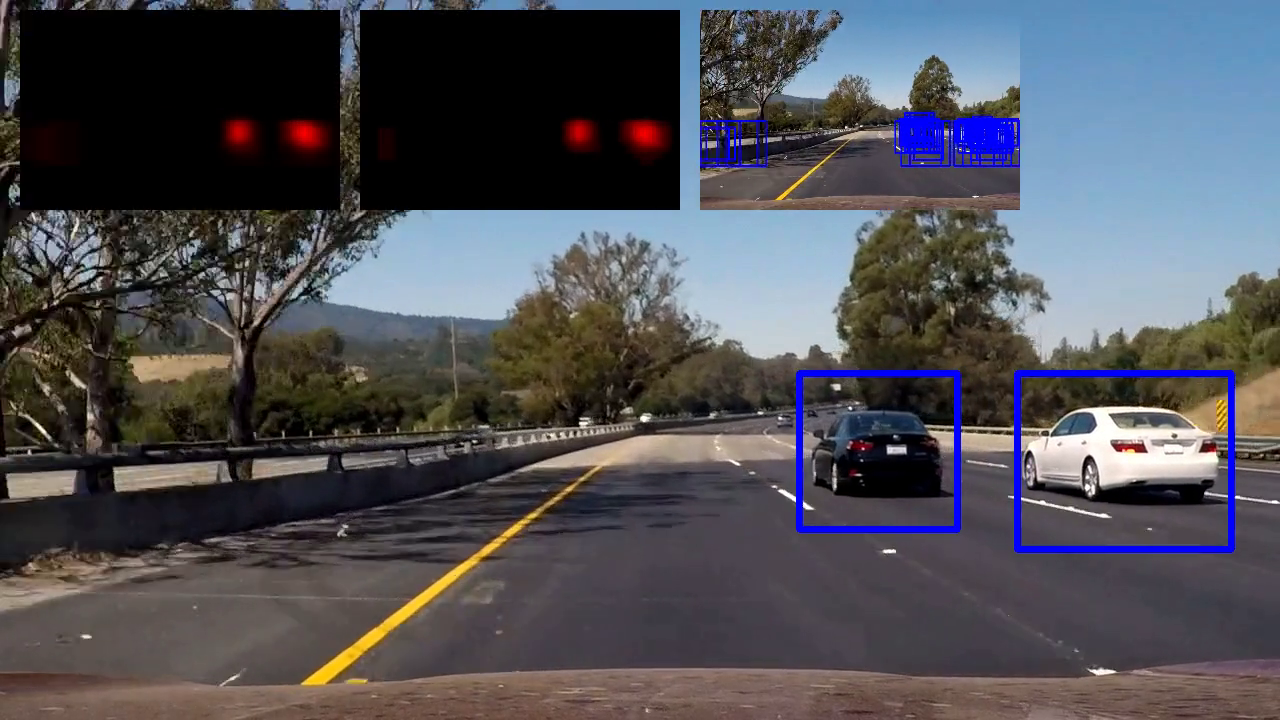There are still many other fields that machine learning can be applied. There is high demand for machine learning engineer today. I believe this is the right time for us to learn about machine learning.

Last Update: Feb 18, 2018.

# Polynomial Curve Fitting

Hi all, after sometimes far from update. I would like give some update today. :D Let’s we discuss about Linear regression. What is linear regression? I would try to explain using curve fitting problem.  Suppose we have 100 pair data, x and t. Where x is the input (1 dimension) and t is the target (1 dimension).

x: {𝑥1,𝑥2,…,𝑥100} represents the input values and
t: {𝑡1,𝑡2,…,𝑡100} represents the target values.

Let us spit them into training set and test set. Training set is the first 80 data and the test set is the last 20 data. We will fit the data by applying the following (model function) polynomial function.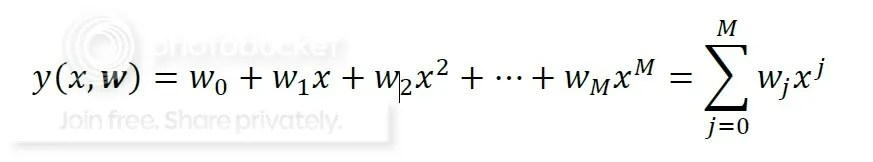The goal is to identify the coefficients w (vector) such that y(x,w) ‘fits’ the data well. The ‘best’ curve has minimum error between curve and data points.  This is called the least squares approach, since we minimize the square of the error. The least squares  as follow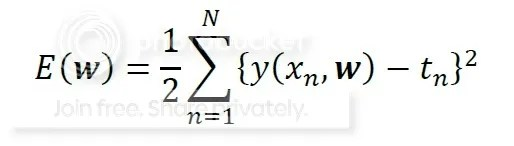This is the plot of original data.